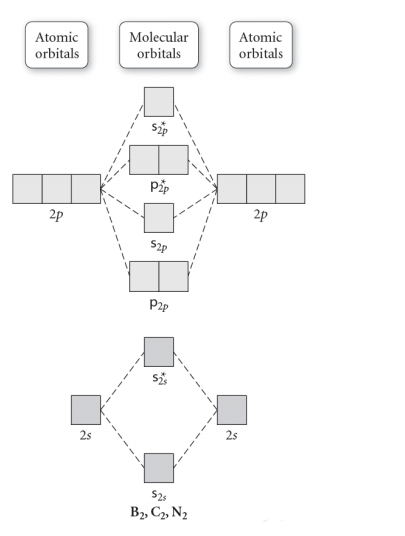# Problem: Use the molecular orbital diagram shown to determine which of the following is most stable.A) N22+ B) B2C) B22+D) C22-E) C22+

###### FREE Expert Solution
93% (470 ratings)
###### FREE Expert Solution

We’re being asked which species is the most stable. For this, we need to determine the bond order for each species. The bond order tells us the stability of a bonda higher bond order means the bond is more stable.

Step 1: Calculate the total number of valence electrons present.

Step 2: Draw the molecular orbital diagram.

Step 3: Calculate the bond order of the molecule/ion. Recall that the formula for bond order is:93% (470 ratings)###### Problem Details

Use the molecular orbital diagram shown to determine which of the following is most stable.

A) N22+

B) B2

C) B22+

D) C22-

E) C22+What scientific concept do you need to know in order to solve this problem?

Our tutors have indicated that to solve this problem you will need to apply the Bond Order concept. If you need more Bond Order practice, you can also practice Bond Order practice problems.

What is the difficulty of this problem?

Our tutors rated the difficulty ofUse the molecular orbital diagram shown to determine which o...as medium difficulty.

How long does this problem take to solve?

Our expert Chemistry tutor, Jules took 4 minutes and 14 seconds to solve this problem. You can follow their steps in the video explanation above.

What professor is this problem relevant for?

Based on our data, we think this problem is relevant for Professor Hoeltge's class at VERMONT.